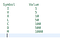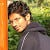# Trello, the perfect tool for productivity

With the increasing seniority in software development, comes up with multiple tasks to manage. I used to struggle between different meetings, completing my tasks, addressing the team’s issues and ad hoc productions bugs. Time used to fly like anything with very less productivity.

Some startups I worked with, did not have a proper tool for bug tracking. They used to create tasks in Google Document or assign bugs on Slack. This created a lot of chaos with no visibility on my work progress. End of the day I did not get the satisfaction of the work being done. …

# Problem statement

Given an integer array nums, find the contiguous subarray (containing at least one number) which has the largest sum and return its sum.

Problem statement taken from: https://leetcode.com/problems/maximum-subarray

Example 1:

`Input: nums = [-2, 1, -3, 4, -1, 2, 1, -5, 4]Output: 6Explanation: [4, -1, 2, 1] has the largest sum = 6.`

Example 2:

`Input: nums = Output: 1`

Example 3:

`Input: nums = [5, 4, -1, 7, 8] Output: 23`

Constraints:

`- 1 <= nums.length <= 3 * 10^4 - -10^5 <= nums[i] <= 10^5`

# Explanation

## Brute force

The brute force approach is to generate all subarrays and…

# Problem statement

Given an array of integers nums sorted in ascending order, find the starting and ending position of a given target value.

If target is not found in the array, return [-1, -1].

Problem statement taken from: https://leetcode.com/problems/find-first-and-last-position-of-element-in-sorted-array

Example 1:

`Input: nums = [5, 7, 7, 8, 8, 10], target = 8Output: [3, 4]`

Example 2:

`Input: nums = [5, 7, 7, 8, 8, 10], target = 6Output: [-1, -1]`

Example 3:

`Input: nums = [], target = 0Output: [-1, -1]`

Constraints:

`- 0 <= nums.length <= 10^5- -10^9 <= nums[i] <= 10^9- nums is a non-decreasing array.…`

# Problem statement

Given a sorted array nums, remove the duplicates in-place such that each element appears only once and returns the new length.

Do not allocate extra space for another array, you must do this by modifying the input array in-place with O(1) extra memory.

Problem statement taken from: https://leetcode.com/problems/remove-duplicates-from-sorted-array

Example 1:

`Input: nums = [1, 1, 2]Output: 2, nums = [1, 2]Explanation: Your function should return length = 2, with the first two elements of nums being 1 and 2 respectively. It doesn't matter what you leave beyond the returned length.`

Example 2:

`Input: nums = [0, 0, 1…`

# Problem statement

Given a non-negative integer x, compute and return the square root of x.

Since the return type is an integer, the decimal digits are truncated, and only the integer part of the result is returned.

Note: You are not allowed to use any built-in exponent function or operator, such as `pow(x, 0.5)` or `x ** 0.5`.

Problem statement taken from: https://leetcode.com/problems/sqrtx

Example 1:

`Input: x = 4Output: 2`

Example 2:

`Input: x = 8 Output: 2 Explanation: The square root of 8 is 2.82842..., and since the decimal part is truncated, 2 is returned.`

Constraints:

`0 <= x <=…`

# Problem statement

Given the head of a linked list, remove the nth node from the end of the list and return its head.

Example 1:

`Input: head = [1,2,3,4,5], n = 2 Output: [1,2,3,5]`

Example 2:

`Input: head = , n = 1Output: []`

Example 3:

`Input: head = [1,2], n = 1 Output: `

Constraints:

`- The number of nodes in the list is sz. - 1 <= sz <= 30 - 0 <= Node.val <= 100 - 1 <= n <= sz`

# Explanation

## Single pointer

One of the approaches to solve this problem is use single pointer by following the below steps

# LeetCode Roman to IntegerImage courtesy: Google

# Problem statement

Roman numerals are represented by seven different symbols: I, V, X, L, C, D and M.

`Symbol       ValueI             1V             5X             10L             50C             100D             500M             1000`

For example, 2 is written as II in Roman numeral, just two one’s added together. 12 is written as XII, which is simply X + II. The number 27 is written as XXVII, which is XX + V + II.

Roman numerals are usually written largest to smallest from left to right. However, the numeral for four is not IIII. Instead, the number four is written as IV

# Problem statement

Given N non-negative integers a1, a2, …, an, where each represents a point at coordinate(i, ai). N vertical lines are drawn such that the two endpoints of the line i is at (i, ai) and (i, 0). Find two lines, which, together with the x-axis forms a container, such that the container contains the most water.

Problem statement taken from: https://leetcode.com/problems/container-with-most-water

Example 1:

`Input: height = [1, 8, 6, 2, 5, 4, 8, 3, 7]Output: 49`

Example 2:

`Input: height = [1, 1] Output: 1`

Example 3:

`Input: height = [4, 3, 2, 1, 4] Output: 16`

Example 4:

`…`

# Problem statement

Given an integer x, return true if x is palindrome integer.

An integer is a palindrome when it reads the same backward as forward. For example, 121 is palindrome while 123 is not.

Example 1:

`Input: x = 121Output: true`

Example 2:

`Input: x = -121 Output: false`

Example 3:

`Input: x = 10Output: false`

Example 4:

`Input: x = -101 Output: false`

Constraints:

`- -2^31 <= x <= 2^31 - 1`

# Explanation

As mentioned in the problem statement, palindrome number is one that reads the same from both sides.

## Brute force solution

A brute force solution will be to convert the…

# LeetCode String to Integer (atoi)

Problem statement

Implement the myAtoi(string s) function, which converts a string to a 32-bit signed integer (similar to C/C++’s atoi function).

Problem statement taken from: https://leetcode.com/problems/string-to-integer-atoi

Example 1:

`Input: s = "42"Output: 42`

Example 2:

`Input: s = "      -142"Output: 142`

Example 3:

`Input: s = "871 and words"Output: 871`

Example 4:

`Input: s = "Words and then number 987"Output: 0`

Example 5:

`Input: s = "-91283472332"Output: -2147483648`

Constraints:

`- 0 <= s.length <= 200- s consists of English letters (lower-case and upper-case), digits (0-9), ' ', '+', '-', and '.'`

Explanation

The problem…## Alkesh Ghorpade

Software Engineer. Working Saeloun. My portfolio https://alkeshghorpade.me